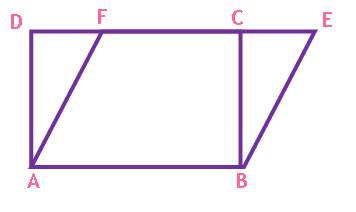Maths / Areas Of Parallelograms And Triangles / Figures on the Same Base and Between the Same Parallels

QUESTION

 In the given figure, ABEF is a parallelogram and ABCD is a rectangle.If EF=x, then CD is equal toOPTIONS A. 2x B. C. x D. x+a
Right Option : C

EXPLANATION
Explain TypeExplanation Content
Text

As parallelogram ABEF and rectangle ABCD are on the same base AB and between the same parallels AB || DE,

AB = EF         opposite sides of parallelogram

AB = CD         opposite sides of rectangle

EF= CD= x

TestimonialsStudent Feedback on Methodology - Prisha Gupta C/o ABHYAS Academy
7th
This academy have a planned schedule and lessons are been taught in such a way that we learn many things to make a base for the next class.